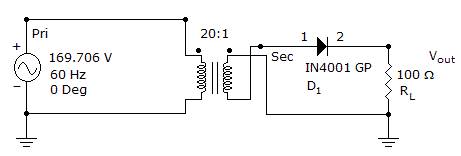# Electronics - Diodes and Applications

### Exercise :: Diodes and Applications - General Questions

31.

With a 12 V supply, a silicon diode, and a 370-ohm resistor in series, what voltage will be dropped across the diode?

 A. 0.3 V B. 0.7 V C. 0.9 V D. 1.4 V

Answer: Option B

Explanation:

No answer description available for this question. Let us discuss.

32.

If the frequency of the applied ac signal to a half-wave rectifier is 60 Hz, the frequency of the pulsating dc output will be

 A. 30 pps B. 60 pps C. 90 pps D. 120 pps

Answer: Option B

Explanation:

No answer description available for this question. Let us discuss.

33.

What is the peak output voltage for this half-wave rectifier?A. 1 V B. 7.8 V C. 10.9 V D. 15.6 V

Answer: Option B

Explanation:

No answer description available for this question. Let us discuss.

34.

Thermal shutdown occurs in an IC regulator if:

 A. power dissipation is too high B. internal temperature is too high C. current through the device is too high D. load resistance increases

Answer: Option B

Explanation:

No answer description available for this question. Let us discuss.

35.

The conduction band is closest to the valence band in

 A. semiconductors B. conductors C. insulators D. The distance is the same for all of the above.

Answer: Option A

Explanation:

No answer description available for this question. Let us discuss.

#### Current Affairs 2021

Interview Questions and Answers